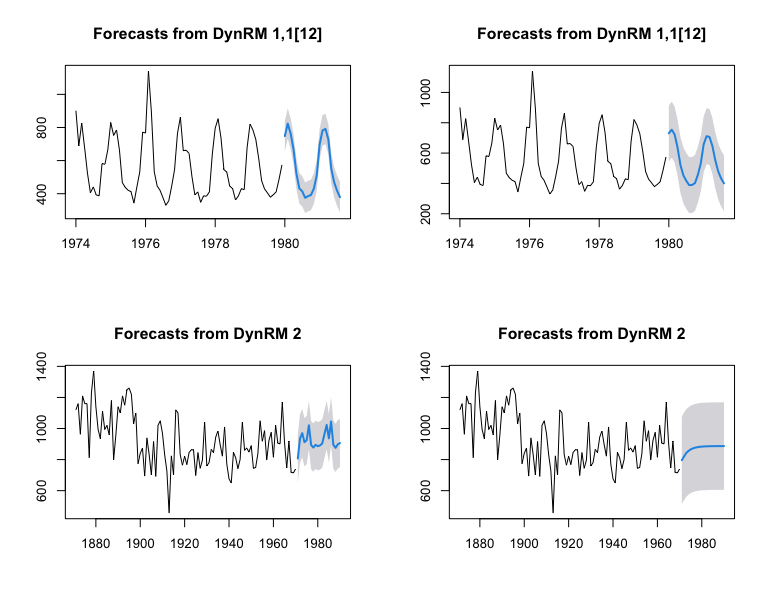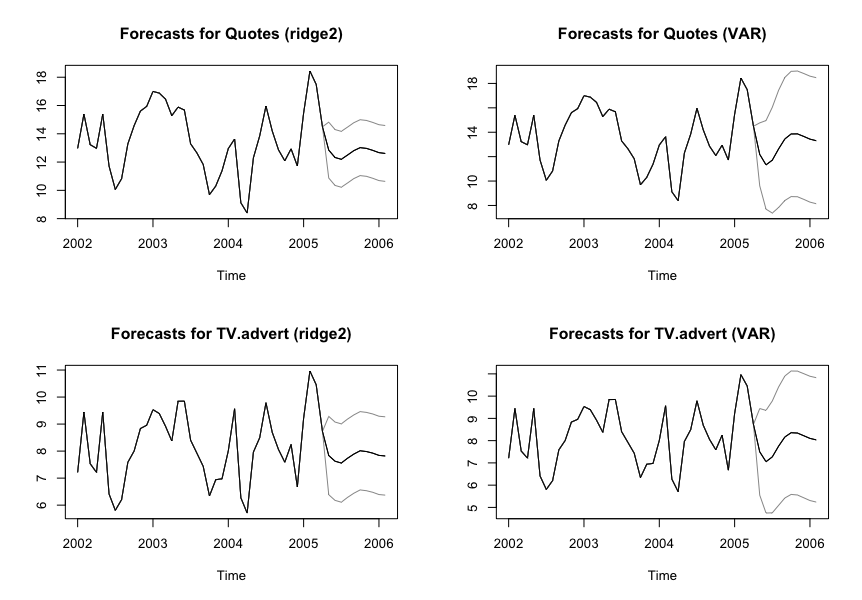A few weeks ago, I introduced a Forecasting API that I deployed on Heroku. Under the hood, this API is built on top of ahead (and through Python packages rpy2 and Flask); an R package for univariate and multivariate time series forecasting. As of October 13th, 2021, 5 forecasting methods are implemented in ahead:

• armagarchf: univariate time series forecasting method using simulation of an ARMA(1, 1) - GARCH(1, 1)
• dynrmf: univariate time series forecasting method adapted from forecast::nnetar to support any Statistical/Machine learning model (such as Ridge Regression, Random Forest, Support Vector Machines, etc.)
• eatf: univariate time series forecasting method based on combinations of forecast::ets, forecast::auto.arima, and forecast::thetaf
• ridge2f: multivariate time series forecasting method, based on quasi-randomized networks and presented in this paper
• varf: multivariate time series forecasting method using Vector AutoRegressive model (VAR, mostly here for benchmarking purpose)

Here’s how to install the package:

• 1st method: from R-universe

In R console:

  options(repos = c(
techtonique = 'https://techtonique.r-universe.dev',
CRAN = 'https://cloud.r-project.org'))


• 2nd method: from Github

In R console:

  devtools::install_github("Techtonique/ahead")


Or

  remotes::install_github("Techtonique/ahead")


And here are the packages that will be used for this demo:

library(ahead)
library(fpp)
library(datasets)
library(randomForest)
library(e1071)


# Univariate time series

In this section, we illustrate dynrmf for univariate time series forecasting, using Random Forest and SVMs. Do not hesitate to type ?dynrmf, ?armagarchf or ?eatf in R console for more details and examples.


par(mfrow=c(2, 2))

# Plotting forecasts
# With a Random Forest regressor, an horizon of 20,
# and a 95% prediction interval
plot(dynrmf(fdeaths, h=20, level=95, fit_func = randomForest::randomForest,
fit_params = list(ntree = 50), predict_func = predict))

# With a Support Vector Machine regressor, an horizon of 20,
# and a 95% prediction interval
plot(dynrmf(fdeaths, h=20, level=95, fit_func = e1071::svm,
fit_params = list(kernel = "linear"), predict_func = predict))

plot(dynrmf(Nile, h=20, level=95, fit_func = randomForest::randomForest,
fit_params = list(ntree = 50), predict_func = predict))

plot(dynrmf(Nile, h=20, level=95, fit_func = e1071::svm,
fit_params = list(kernel = "linear"), predict_func = predict))# Multivariate time series

In this section, we illustrate ridge2f and varf forecasting for multivariate time series. Do not hesitate to type ?ridge2f or ?varf in R console for more details on both functions.

# Forecast using ridge2
# With 2 time series lags, an horizon of 10,
# and a 95% prediction interval
fit_obj_ridge2 <- ahead::ridge2f(fpp::insurance, lags = 2,
h = 10, level = 95)

# Forecast using VAR
fit_obj_VAR <- ahead::varf(fpp::insurance, lags = 2,
h = 10, level = 95)

# Plotting forecasts
# fpp::insurance contains 2 time series, Quotes and TV.advert
par(mfrow=c(2, 2))
plot(fit_obj_ridge2, "Quotes")
plot(fit_obj_VAR, "Quotes")